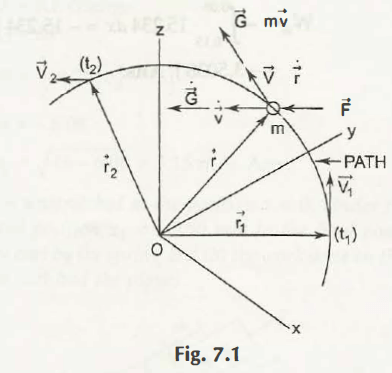Linear momentum, sometimes simply call as momentum is the product of mass of the object and its velocity in the given direction. Linear momentum is a vector quantity and its direction will be same as the velocity of the object.

It can be formulated as-

G ⃗    =    mV ⃗

Fig. 7.1 shows the linear momentum of an object about a point ODifferentiation the above equation, we get-

(dG ⃗)/dx    =    m (dV ⃗)/dx
=    m a ⃗
(dG ⃗)/dx    =    ∑▒□(F ⃗ )
Or,
∑▒□(F ⃗ )    =    G ̇  ⃗

Where,
∑▒□(F ⃗ )= Resultant of forces. It can be calculated as-
Resultant Force,
∑▒□(F ⃗ )= ∑▒Fxi ̂ +∑▒Fyj ̂+ ∑▒Fzk ̂
= G ̇xi ̂ +G ̇yj ̂+ G ̇zk ̂
Or,
∑▒Fx = G ̇x
∑▒Fy= G ̇y
∑▒Fz= G ̇z

Note:
The S.I. unit of Linear Momentum is kg. m/s

Links of Next Mechanical Engineering Topics:-### Customer Reviews

My Homework Help
Rated 5.0 out of 5 based on 510 customer reviews at